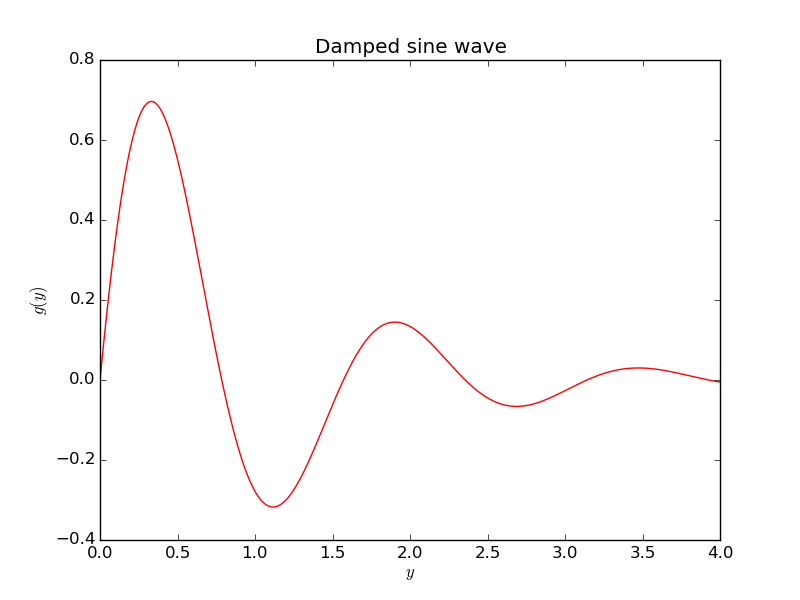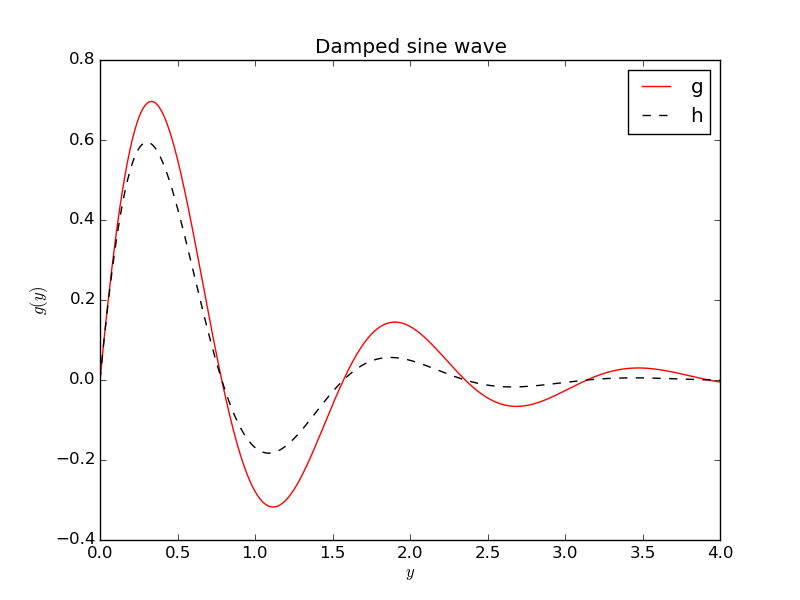# Exercises

## Exercise 1: Program a formula

a) Make a simplest possible Python program that calculates and prints the value of the formula $$y = 6x^2 + 3x + 2,\] for x=2.$$

x = 2
y = 6*x**2 + 3*x + 2
print(y)


b) Make a Python function that takes $$x$$ as argument and returns $$y$$. Call the function for $$x=2$$ and print the answer.

Code:

def f(x):
return 6*x**2 + 3*x + 2

y = f(2)
print(y)


## Exercise 2: Combine text and numbers in output

Let $$y=x^2$$. Make a program that writes the text

y(2.550)=6.502


if $$x=2.55$$. The values of $$x$$ and $$y$$ should be written with three decimals. Run the program for $$x=\pi$$ too (the value if $$\pi$$ is available as the variable pi in the math module).

Here is the code:

x = 2.55
y = x**2
print('y(%.3f)=%.3f' % (x, y))


Changing $$x=2.55$$ to $$x=\pi$$,

from math import pi
x = pi
y = x**2
print('y(%.3f)=%.3f' % (x, y))


gives the output y(3.142)=9.870.

## Exercise 3: Program a while loop

Define a sequence of numbers, $$x_n = n^2 + 1,$$ for integers $$n=0,1,2,\ldots,N$$. Write a program that prints out $$x_n$$ for $$n=0,1,\ldots,20$$ using a while loop.

Complete program:

n = 0
while n <= 20:
x_n = n**2 + 1
print('x%d=%d' % (n, x_n))
n = n + 1


## Exercise 4: Create a list with a while loop

Store all the $$x_n$$ values computed in Exercise 3: Program a while loop in a list (using a while loop). Print the entire list (as one object).

Code:

n = 0
x = []  # the x_n values
while n <= 20:
x.append(n**2 + 1)
n = n + 1
print(x)


## Exercise 5: Program a for loop

Do Exercise 4: Create a list with a while loop, but use a for loop.

Code:

x = []
for n in range(21):
x.append(n**2 + 1)
print(x)


One can also make the code shorter using a list comprehension:

x = [n**2 + 1 for n in range(21)]
print(x)


## Exercise 6: Write a Python function

Write a function x(n) for computing an element in the sequence $$x_n=n^2+1$$. Call the function for $$n=4$$ and write out the result.

Code:

def x(n):
return n^2 + 1

print(x(4))


## Exercise 7: Return three values from a Python function

Write a Python function that evaluates the mathematical functions $$f(x)=\cos(2x)$$, $$f'(x)=-2\sin(2x)$$, and $$f''(x)=-4\cos(2x)$$. Return these three values. Write out the results of these values for $$x=\pi$$.

Code:

from math import sin, cos, pi

def deriv2(x):
return cos(2*x), -2*sin(2*x), -4*cos(2*x)

f, df, d2f = deriv2(x=pi)
print(f, df, d2f)


Running the program gives

Terminal> python deriv2.py
1.0 4.89858719659e-16 -4.0


as expected.

## Exercise 8: Plot a function

Make a program that plots the function $$g(y)=e^{-y}\sin (4y)$$ for $$y\in [0,4]$$ using a red solid line. Use 500 intervals for evaluating points in $$[0,4]$$. Store all coordinates and values in arrays. Set labels on the axis and use a title "Damped sine wave".

Appropriate code is

import numpy as np
import matplotlib.pyplot as plt
from numpy import exp, sin  # avoid np. prefix in g(y) formula

def g(y):
return exp(-y)*sin(4*y)

y = np.linspace(0, 4, 501)
values = g(y)
plt.figure()
plt.plot(y, values, 'r-')
plt.xlabel('$y$'); plt.ylabel('$g(y)$')
plt.title('Damped sine wave')
plt.savefig('tmp.png'); plt.savefig('tmp.pdf')
plt.show()## Exercise 9: Plot two functions

As Exercise 9: Plot two functions, but add a black dashed curve for the function $$h(y)=e^{-\frac{3}{2}y}\sin (4y)$$. Include a legend for each curve (with names g and h).

Here is the program:

import numpy as np
import matplotlib.pyplot as plt
from numpy import exp, sin  # avoid np. prefix in g(y) and h(y)

def g(y):
return exp(-y)*sin(4*y)

def h(y):
return exp(-(3./2)*y)*sin(4*y)

y = np.linspace(0, 4, 501)
plt.figure()
plt.plot(y, g(y), 'r-', y, h(y), 'k--')
plt.xlabel('$y$'); plt.ylabel('$g(y)$')
plt.title('Damped sine wave')
plt.legend(['g', 'h'])
plt.savefig('tmp.png'); plt.savefig('tmp.pdf')
plt.show()## Exercise 10: Measure the efficiency of vectorization

IPython an enhanced interactive shell for doing computing with Python. IPython has some user-friendly functionality for quick testing of the efficiency of different Python constructions. Start IPython by writing ipython in a terminal window. The interactive session below demonstrates how we can use the timer feature %timeit to measure the CPU time required by computing $$\sin (x)$$, where $$x$$ is an array of 1M elements, using scalar computing with a loop (function sin_func) and vectorized computing using the sin function from numpy.

In : import numpy as np

In : n = 1000000

In : x = np.linspace(0, 1, n+1)

In : def sin_func(x):
...:     r = np.zeros_like(x)  # result
...:     for i in range(len(x)):
...:         r[i] = np.sin(x[i])
...:     return r
...:

In : %timeit y = sin_func(x)
1 loops, best of 3: 2.68 s per loop

In : %timeit y = np.sin(x)
10 loops, best of 3: 40.1 ms per loop


Here, %timeit ran our function once, but the vectorized function 10 times. The most relevant CPU times measured are listed, and we realize that the vectorized code is $$2.68/(40.1/1000)\approx 67$$ times faster than the loop-based scalar code.

Use the recipe above to investigate the speed up of the vectorized computation of the $$s(t)$$ function in the section Functions.